# 不懂动态规划？21道 LeetCode题目带你学会动态规划！

“这是我参与8月更文挑战的第17天，活动详情查看： 8月更文挑战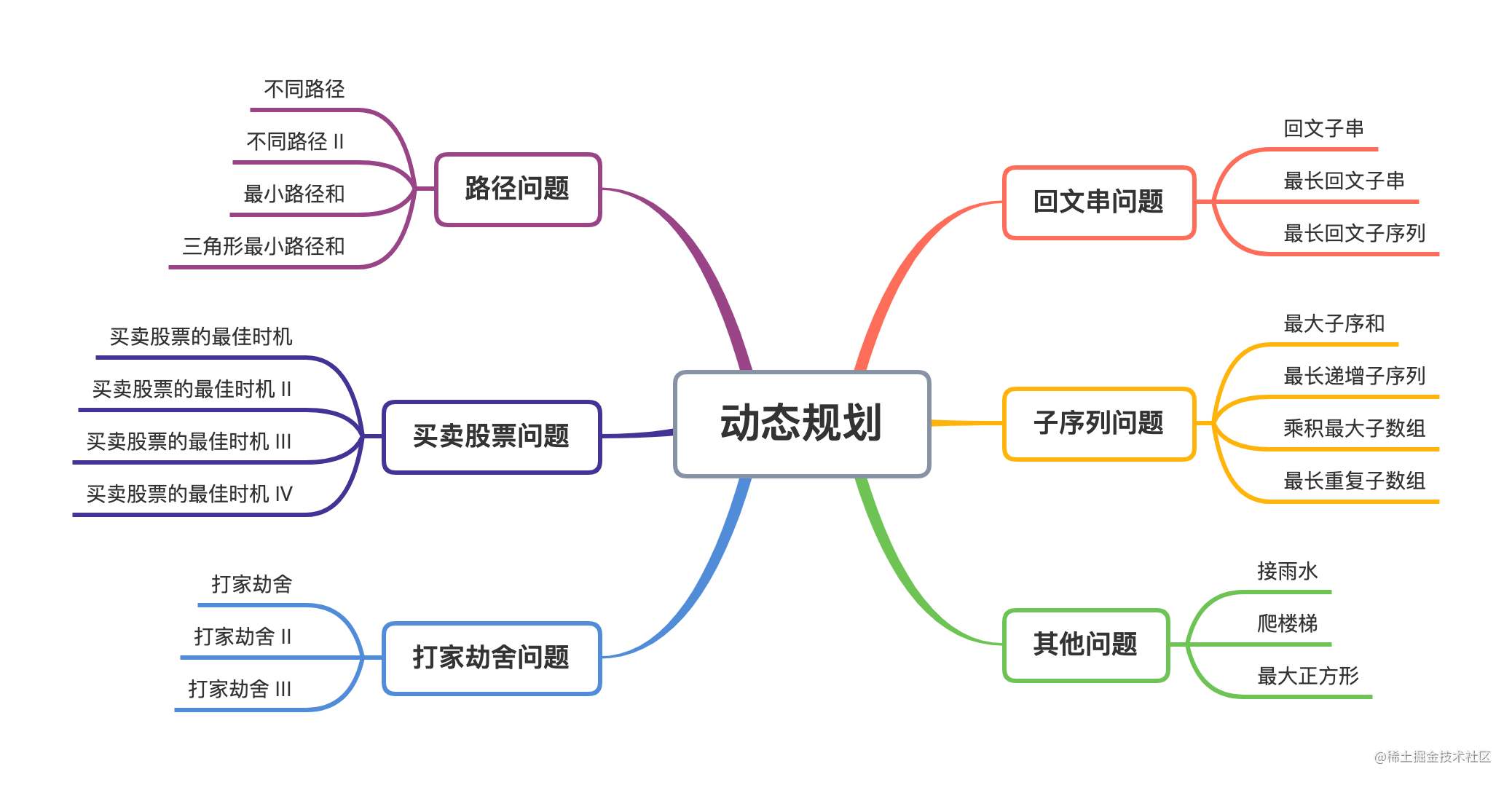### 1. 动态规划概述

#### （1）基本概念

• 状态：解决某一问题的中间结果，它是子问题的一个抽象定义。
• 状态转移方程：状态与状态之间的递推关系。

1. 状态定义：找出子问题抽象定义。
2. 确定状态转移方程：找出状态与状态之间的递推关系。
3. 初始状态和边界情况：最简单的子问题的结果，也是程序的出口条件 。
4. 返回值：对于简单问题，返回值可能就是最终状态；对于复杂问题可能还需对最终状态做一些额外处理。

``````输入： 2

1. 1 阶 + 1 阶
2. 2 阶

``````输入： 3

1. 1 阶 + 1 阶 + 1 阶
2. 1 阶 + 2 阶
3. 2 阶 + 1 阶

• 要求给出达成某个目的的解法个数；
• 不要求给出每一种解法对应的具体路径。

• 最后一步爬 1 级台阶，前面有 n - 1 级台阶，这种情况下共有f(n - 1)种方法；
• 最后一步爬 2 级台阶，前面有 n - 2 级台阶，这种情况下共有f(n - 2)种方法；

f(n) 为以上两种情况之和，即 f(n)=f(n-1)+f(n-2)，这就是本题用到的递推关系。下面就根据动态规划的四个步骤来看那一下：

1. 状态定义：初始化一个f数组，f[i]表示爬到i级台阶的方法数量；
2. 状态转移方程：f(n)=f(n-1)+f(n-2)；
3. 初始状态：一级台阶时，共1种爬法；两级台阶时，可以一级一级爬，也可以一次爬两级，共有2种爬法。即f = 1，f = 2；
4. 返回值：f[n] ，即 n 级台阶共有多少种爬法。

``````/**
* @param {number} n
* @return {number}
*/
const climbStairs = function(n) {
// 初始化状态数组
const f = [];
// 初始化已知值
f = 1;
f = 2;
// 动态更新每一层楼梯对应的结果
for(let i = 3;i <= n;i++){
f[i] = f[i-2] + f[i-1];
}
// 返回目标值
return f[n];
};

#### （2）使用场景

• 最优子结构，它指的是问题的最优解包含着子问题的最优解——不管前面的决策如何，此后的状态必须是基于当前状态（由上次决策产生）的最优决策。就这道题来说，`f(n)``f(n-1)``f(n-2)`之间的关系（状态转移方程）印证了这一点。
• 重叠子问题，在递归的过程中，出现了反复计算的情况。
• 无后效性，无后效性有两层含义，第一层含义是，在推导后面阶段的状态的时候，只关心前面阶段的状态值，不关心这个状态是怎么一步一步推导出来的。第二层含义是，某阶段状态一旦确定，就不受之后阶段的决策影响。无后效性是一个非常“宽松”的要求。只要满足前面提到的动态规划问题模型，其实基本上都会满足无后效性。

### 2. LeetCode 路径问题

#### （1）不同路径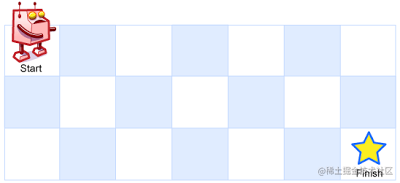``````输入：m = 3, n = 7

``````输入：m = 3, n = 2

1. 向右 -> 向下 -> 向下
2. 向下 -> 向下 -> 向右
3. 向下 -> 向右 -> 向下

``````输入：m = 7, n = 3

``````输入：m = 3, n = 3

• `1 <= m, n <= 100`
• 题目数据保证答案小于等于 `2 * 10`

``````a[i][j] = a[i - 1][j] + a[i][j - 1]

``````/**
* @param {number} m
* @param {number} n
* @return {number}
*/
var uniquePaths = function(m, n) {
const dp = new Array(m).fill(0).map(() => new Array(n).fill(0))

for(let i = 0; i < m; i++){
dp[i] = 1
}
for(let j = 0; j < n; j++){
dp[j] = 1
}

for(let i = 1; i < m; i++){
for(let j = 1; j < n; j++){
dp[i][j] = dp[i - 1][j] + dp[i][j - 1]
}
}
return dp[m - 1][n - 1]
};

• 时间复杂度：O(mn)，其中m和n分别是网格的长宽，我们需要两层遍历，所以空间复杂度为O(mn)。
• 空间复杂度：O(mn)，其中m和n分别是网格的长宽，我们需要一个m * n 的二维数组来存储所有状态，所以所需空间复杂度为O(mn)。

#### （2）不同路径 II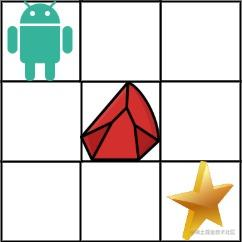``````输入：obstacleGrid = [[0,0,0],[0,1,0],[0,0,0]]

3x3 网格的正中间有一个障碍物。

1. 向右 -> 向右 -> 向下 -> 向下
2. 向下 -> 向下 -> 向右 -> 向右``````输入：obstacleGrid = [[0,1],[0,0]]

• `m == obstacleGrid.length`
• `n == obstacleGrid[i].length`
• `1 <= m, n <= 100`
• `obstacleGrid[i][j]``0``1`

• 在给第一行和第一列元素设置初始值时，如果遇到网格的值是1，也就是有障碍物的情况，就直接停下来，不需要往前继续遍历了，因为前面就不可能在经过了；
• 在计算每个网格的路径数时，如果该方格元素是就直接跳过，不需要计算。

``````/**
* @param {number[][]} obstacleGrid
* @return {number}
*/
var uniquePathsWithObstacles = function(obstacleGrid) {
if(!obstacleGrid.length || obstacleGrid === 1){
return 0
}

const m = obstacleGrid.length, n = obstacleGrid.length
const dp = new Array(m).fill(0).map(() =>  new Array(n).fill(0))

for (let i = 0; i < m && obstacleGrid[i] == 0; i++) {
dp[i] = 1;
}
for (let j = 0; j < n && obstacleGrid[j] == 0; j++) {
dp[j] = 1;
}

for(let i = 1; i < m; i++){
for(let j = 1; j < n; j++){
if(obstacleGrid[i][j] === 0){
dp[i][j] = dp[i - 1][j] + dp[i][j - 1]
}
}
}
return dp[m - 1][n - 1]
};

• 时间复杂度：O(mn)，其中m和n分别是网格的长宽，我们需要两层遍历，所以空间复杂度为O(mn)。
• 空间复杂度：O(mn)，其中m和n分别是网格的长宽，我们需要一个m * n 的二维数组来存储所有状态，所以所需空间复杂度为O(mn)。

#### （3）最小路径和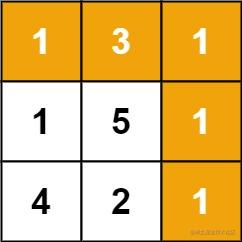``````输入：grid = [[1,3,1],[1,5,1],[4,2,1]]

``````输入：grid = [[1,2,3],[4,5,6]]

• `m == grid.length`
• `n == grid[i].length`
• `1 <= m, n <= 200`
• `0 <= grid[i][j] <= 100`

``````grid[i] += grid[i - 1]

``````grid[j] += grid[j - 1]

``````grid[i][j] = Math.min(grid[i - 1][j], grid[i][j - 1]) + grid[i][j];

``````/**
* @param {number[][]} grid
* @return {number}
*/
var minPathSum = function(grid) {
let m = grid.length, n = grid.length

for(let i = 1; i < m; i++){
grid[i] += grid[i - 1]
}
for(let j = 1; j < n; j++){
grid[j] += grid[j - 1]
}

for(let i = 1; i < m; i++){
for(let j = 1; j < n; j++){
grid[i][j] = Math.min(grid[i - 1][j], grid[i][j - 1]) + grid[i][j];
}
}
return grid[m - 1][n - 1]
}

• 时间复杂度：O(mn)，其中 m 和 n 分别是网格的行数和列数。需要对整个网格遍历一次，计算 grid 的每个元素的值。
• 空间复杂度：O(1)，这里我们是在原数组的基础上进行的操作，所需的额外的空间为常数。

#### （4）三角形最小路径和

``````输入：triangle = [,[3,4],[6,5,7],[4,1,8,3]]

2
3 4
6 5 7
4 1 8 3

``````输入：triangle = [[-10]]

• `1 <= triangle.length <= 200`
• `triangle.length == 1`
• `triangle[i].length == triangle[i - 1].length + 1`
• `-104 <= triangle[i][j] <= 104`

进阶：

• 你可以只使用 `O(n)` 的额外空间（`n` 为三角形的总行数）来解决这个问题吗？

``````triangle[i][j] += triangle[i - 1][j]

``````triangle[i][j] += triangle[i - 1][j - 1]

``````triangle[i][j] += Math.min(triangle[i - 1][j], triangle[i - 1][j - 1])

``````/**
* @param {number[][]} triangle
* @return {number}
*/
var minimumTotal = function(triangle) {
const n = triangle.length

for(let i = 1; i < n; i++){
for(let j = 0; j <= i; j++){
if(j === 0){
triangle[i][j] += triangle[i - 1][j]
}else if(j === i){
triangle[i][j] += triangle[i - 1][j - 1]
}else{
triangle[i][j] += Math.min(triangle[i - 1][j], triangle[i - 1][j - 1])
}
}
}

return Math.min(...triangle[n - 1])
};

• 时间复杂度：O(n2)，其中 n 是三角形的行数。
• 空间复杂度：O(1)。这里我们在原数组的基础上进行的操作，所以所需要的额外的空间为常数。

### 3. LeetCode 买卖股票问题

#### （1）买卖股票的最佳时机

``````输入: [7,1,5,3,6,4]

注意利润不能是 7-1 = 6, 因为卖出价格需要大于买入价格；同时，你不能在买入前卖出股票。

``````输入: [7,6,4,3,1]

（1）直接遍历

（2）动态规划

• `dp`：一直没有买
• `dp`：：到最后只买了一笔，未卖出
• `dp`：：到最后只卖了一笔，并卖出

• `dp = Math.max(dp, -prices[i])`：前一天也是b1状态或者是没有任何操作，今天买入一笔变成b1状态；
• `dp = Math.max(dp, dp + prices[i])`：前一天也是s1状态或者是b1状态，今天卖出一笔变成s1状态；

（1）直接遍历

``````/**
* @param {number[]} prices
* @return {number}
*/
var maxProfit = function(prices) {
let max = 0
let min = prices

prices.forEach( item => {
if(item < min) min = item
if(item - min > max) max = item - min
})
return max
};

• 时间复杂度： O(n)，其中n是数组的长度，我们需要将数组遍历一遍
• 空间复杂度： O(1)，这里只需要常数空间来储存最小值min和最大结果值max

（2）动态规划

``````/**
* @param {number[]} prices
* @return {number}
*/
var maxProfit = function(prices) {
let len = prices.length;
const dp = [0, -prices, 0]

for (let i = 1; i < len; i++) {
dp = Math.max(dp, -prices[i])
dp = Math.max(dp, dp + prices[i])
}
return dp;
}

• 时间复杂度：O(n)，其中 n 是数组 prices 的长度。
• 空间复杂度：O(1)。

#### （2）买卖股票的最佳时机 II

``````输入: [7,1,5,3,6,4]

随后，在第 4 天（股票价格 = 3）的时候买入，在第 5 天（股票价格 = 6）的时候卖出, 这笔交易所能获得利润 = 6-3 = 3 。

``````输入: [1,2,3,4,5]

注意你不能在第 1 天和第 2 天接连购买股票，之后再将它们卖出。
因为这样属于同时参与了多笔交易，你必须在再次购买前出售掉之前的股票。

``````输入: [7,6,4,3,1]

• 1 <= prices.length <= 3 * 10
• 0 <= prices[i] <= 10

1）dp[i]表示：第 i 天手里没股票，至今（第 i 天）的最大收益。第 i 天手里没股票，有两种可能：

• 昨天也没持有股票：dp[i-1]
• 昨天买了股票，今天卖了: dp[i-1] + prices[i]
• dp[i] = max(dp[i-1], dp[i-1] + prices[i])

2）dp[i]表示：第 i 天手里有股票，至今（第 i 天）的最大收益。第 i 天手里有股票，有两种可能：

• 昨天也有股票：dp[i-1]
• 昨天卖了，今天买了: dp[i-1] - prices[i]
• dp[i] = max(dp[i-1], dp[i-1] - prices[i])

• day 0 没买:dp = 0
• day 0 买了:dp = -prices
``````/**
* @param {number[]} prices
* @return {number}
*/
function maxProfit(prices) {
const len = prices.length;
if (len < 2) {
return 0;
};
const dp = new Array(len);
dp = [0, -prices];
for (let i = 1; i < len; i++) {
dp[i] = new Array(2);
dp[i] = Math.max(dp[i - 1], dp[i - 1] + prices[i]); // 没有股票
dp[i] = Math.max(dp[i - 1], dp[i - 1] - prices[i]); // 有股票
}
return dp[len - 1];
}

• 时间复杂度： O(n)，其中 n 为数组的长度。一共有 2n 个状态，每次状态转移的时间复杂度为 O(1)，因此时间复杂度为 O(2n)=O(n)。
• 空间复杂度：O(n)，我们需要开辟O(n) 空间存储动态规划中的所有状态。

#### （3）买卖股票的最佳时机 III

``````输入：prices = [3,3,5,0,0,3,1,4]

随后，在第 7 天（股票价格 = 1）的时候买入，在第 8 天 （股票价格 = 4）的时候卖出，这笔交易所能获得利润 = 4-1 = 3 。

``````输入：prices = [1,2,3,4,5]

注意你不能在第 1 天和第 2 天接连购买股票，之后再将它们卖出。
因为这样属于同时参与了多笔交易，你必须在再次购买前出售掉之前的股票。

``````输入：prices = [7,6,4,3,1]

``````输入：prices = 

• 1 <= prices.length <= 105
• 0 <= prices[i] <= 105

• `dp`：一直没有买
• `dp`：到最后只买了一笔，未卖出
• `dp`：到最后只卖了一笔，并卖出
• `dp`：到最后买了两笔，只卖出一笔
• `dp`：到最后买了两笔，两笔都卖出

• `dp = Math.max(dp, -prices[i])`：前一天也是b1状态或者是没有任何操作，今天买入一笔变成b1状态；
• `dp = Math.max(dp, dp + prices[i])`：前一天也是s1状态或者是b1状态，今天卖出一笔变成s1状态；
• `dp = Math.max(dp, dp - prices[i])`：前一天也是b2状态或者是s1状态，今天买入一笔变成b2状态；
• `dp = Math.max(dp, dp + prices[i])`：前一天也是s2状态或者是b2状态，今天冒出一笔变成s2状态。
``````/**
* @param {number[]} prices
* @return {number}
*/
function maxProfit(prices) {
let len = prices.length;
const dp = [0, -prices, -prices, 0, 0]

for (let i = 1; i < len; i++) {
dp = Math.max(dp, -prices[i])
dp = Math.max(dp, dp + prices[i])
dp = Math.max(dp, dp - prices[i])
dp = Math.max(dp, dp + prices[i])
}
return dp;
};

• 时间复杂度：O(n)，其中 n 是数组 prices 的长度。
• 空间复杂度：O(1)。

#### （4）买卖股票的最佳时机 IV

``````输入：k = 2, prices = [2,4,1]

``````输入：k = 2, prices = [3,2,6,5,0,3]

随后，在第 5 天 (股票价格 = 0) 的时候买入，在第 6 天 (股票价格 = 3) 的时候卖出, 这笔交易所能获得利润 = 3-0 = 3 。

• 0 <= k <= 100
• 0 <= prices.length <= 1000
• 0 <= prices[i] <= 1000

• 无操作，一直没有买
• dp：到最后只买了一笔，未卖出
• dp：到最后只卖了一笔，并卖出
• dp：到最后买了两笔，只卖出一笔
• dp：到最后买了两笔，两笔都卖出
• dp：到最后买了三笔，只卖出两笔
• ······

• 不交易，现金 不变
• 进行`[1, k]`的某次交易
• 买入，现金 -= 当天股票价格
• 卖出，现金 += 当天股票价格
``````/**
* @param {number} k
* @param {number[]} prices
* @return {number}
*/
var maxProfit = function(k, prices) {
const dp = new Int16Array(k * 2).fill(-prices)

for (let i = 0; i < prices.length; i++) {
for (let j = 0; j < dp.length; j++)
{
dp[j] = Math.max(dp[j], (dp[j - 1] || 0) + (j & 1 ? prices[i] : -prices[i]))
}
}
return Math.max(0, ...dp)
};

• 时间复杂度：O(n * min(n, k))，其中 n 是数组 prices 的长度，即使用双重循环进行动态规划需要的时间。
• 空间复杂度：O(min(n,k))。

### 4. LeetCode 打家劫舍问题

#### （1）打家劫舍

``````输入：[1,2,3,1]

偷窃到的最高金额 = 1 + 3 = 4 。

``````输入：[2,7,9,3,1]

偷窃到的最高金额 = 2 + 9 + 1 = 12 。

• `0 <= nums.length <= 100`
• `0 <= nums[i] <= 400`

• 如果偷第n个房间，那么就不能偷第n - 1个房间，那么总金额就是前n - 2间屋子能偷到的最高的金额之和；
• 如果不偷第k间屋，那么能偷到的总金额就是前k - 1个房间的最高总金额。

``````dp[i]=max(dp[i−2]+nums[i],dp[i−1])

• dp = nums ：只有一间房屋，则偷窃该房屋
• dp = max(nums, nums)：只有两间房屋，选择其中金额较高的房屋进行偷窃

``````/**
* @param {number[]} nums
* @return {number}
*/
var rob = function(nums) {
const len = nums.length
if(!len){
return 0
}

const dp = new Array(len + 1)
dp = 0
dp = nums

for(let i = 2; i <= len; i++){
dp[i] = Math.max(dp[i-1], dp[i-2] + nums[i-1]);
}
return dp[len];
};

• 时间复杂度：O(n)，其中 n 是数组长度。只需要对数组遍历一次。
• 空间复杂度：O(1)。使用数组只存储前两间房屋的最高总金额，而不需要存储整个数组的结果，因此空间复杂度是 O(1)

#### （2）打家劫舍 II

``````输入：nums = [2,3,2]

``````输入：nums = [1,2,3,1]

偷窃到的最高金额 = 1 + 3 = 4 。

``````输入：nums = 

• `1 <= nums.length <= 100`
• `0 <= nums[i] <= 1000`

• 不偷第一家
• 不偷最后一家

``````dp[i] = Math.max(dp[i - 1], dp[i - 2] + nums[i])

``````/**
* @param {number[]} nums
* @return {number}
*/
var rob = function(nums) {
const len = nums.length
let res1 = 0, res2 = 0
if(len === 0) return 0
if(len === 1) return nums

const dp = new Array(len)

// 不偷第一家
dp = 0
dp = nums
for(let i = 2; i <= len - 1; i++){
dp[i] = Math.max(dp[i - 1], dp[i - 2] + nums[i]);
}
res1 = dp[len - 1]

// 不偷最后一家
dp = nums
dp = Math.max(nums, nums)
for(let i = 2; i <= len - 2; i++){
dp[i] = Math.max(dp[i - 1], dp[i - 2] + nums[i]);
}
res2 = dp[len - 2]
return Math.max(res1, res2)
};

• 时间复杂度：O(n)，其中n是数组的长度，我们需要遍历两次数组；
• 空间复杂度：O(n)，其中n是数组的长度，我们需要初始化一个长度为n的数组来保存当前节点的状态。

#### （3）打家劫舍 III

``````输入: [3,2,3,null,3,null,1]
3
/ \
2   3
\   \
3   1

``````输入: [3,4,5,1,3,null,1]
3
/ \
4   5
/ \   \
1   3   1

• 当节点被选中时，它的左右孩子都不能被选中，所以最大值就是：node.val + left + right；
• 当节点不被选中时，它的左右子孩子可以选中也可以不选中，所以最大值就是：Math.max(left, left) + Math.max(right, right);

``````/**
* Definition for a binary tree node.
* function TreeNode(val, left, right) {
*     this.val = (val===undefined ? 0 : val)
*     this.left = (left===undefined ? null : left)
*     this.right = (right===undefined ? null : right)
* }
*/
/**
* @param {TreeNode} root
* @return {number}
*/
var rob = function(root) {
const dfs = (node) => {
if (node === null) {
return [0, 0];
}
const left = dfs(node.left);
const right = dfs(node.right);

const select = node.val + left + right;
const notSelect = Math.max(left, left) + Math.max(right, right);
return [select, notSelect];
}
const res = dfs(root)
return Math.max(res, res)
};

• 时间复杂度：O(n)，对二叉树进行了一次后序遍历，所以时间复杂度是 O(n)；
• 空间复杂度：O(n)，递归栈空间的使用代价是 O(n)。

### 5. LeetCode 回文串问题

#### （1）回文子串

``````输入："abc"

``````输入："aaa"

• 首先，根据题目，我们可以看出，每个元素自身也算是一个回文子串，所以要将字符串的长度加进去
• 定义一个函数用来检测字符串是否是回文串
• 两层遍历字符串，截取字符串的所有的子串，并判断其是否是回文串

• 时间复杂度为O(n2)，需要两层遍历。
• 空间复杂度为O(1)
``````/**
* @param {string} s
* @return {number}
*/
var countSubstrings = function(s) {
let count = s.length
let len = s.length
for(let i = 0; i < len; i++){
for(let j = i + 1; j < len; j++){
let temp = s.substr(i, j-i+1)
if(isSub(temp)){
count++
}
}
}
return count
};
const isSub = str => {
let a = 0
let b = str.length - 1
while(a <= b){
if(str[a] !== str[b]){
return false
}
a++
b--
}
return true
}

#### （2）最长回文子串

``````输入: "babad"

``````输入: "cbbd"

（1）方法一：中心扩展法

• 选取对称中心（奇数长度的字符串为中心两个字符的中间，偶数长度的字符串中心为中间的字符）
• 通过对比扩展之后得出的两种组合较大的回文子串长度
• 对比之前的长度，判断是否更新起始的位置
• 遍历完之后，根据起始位置，截取最长回文子串

``````/**
* @param {string} s
* @return {string}
*/
var longestPalindrome = function(s) {
if(s == null || s.length <1){
return ''
}
let start = 0
let end = 0
// 定义中心扩展的方法
const fn = (s,left,right) => {
while(left >=0 && right< s.length && s[left] === s[right]){
left--
right++
}
return right - left -1
}
// 遍历字符串
for(let i = 0; i<s.length; i++){
const len1 = fn(s, i, i)
const len2 = fn(s, i, i+1)
const len = Math.max(len1, len2)
// 判断起始位置是否更新
if(len > end - start){
start = i- Math.floor((len-1)/2)
end = i+ Math.floor(len/2)
}
}
return s.substring(start, end+1)
};

• 时间复杂度：O(n2)，枚举“中心位置”时间复杂度为 O(n)，从“中心位置”扩散得到“回文子串”的时间复杂度为 O(n)，因此时间复杂度是 O(n2)。
• 空间复杂度：O(1)，这里只用到了两个常数临时变量start、end，因此空间复杂度为O(1)。

（2）方法二：动态规划

• 如果一个字符串是回文串，那么在它左右分别加上一个相同的字符，那么它一定还是一个回文串
• 如果在一个不是回文字符串的字符串两端添加任何字符，或者在回文串左右分别加不同的字符，得到的一定不是回文串

``````if (s[i] === s[j] && dp[i + 1][j - 1]) {
dp[i][j] = true;
}

• s[i] === s[j]：说明当前中心可以继续扩张，进而有可能扩大回文串的长度
• dp[i+1][j-1]：true，说明s[i,j]的子串s[i+1][j-1]也是回文串，其中，i是从最大值开始遍历的，j是从最小值开始遍历的

• 确定dp[i][j]是否是回文数，只需要dp[i+1][j-1]是回文数并且s[i] === s[j]即可。
• 长度为0或1的回文传需要特殊处理，即j-i < 2;
• 因为知道dp[i]需要先知道dp[i+1]，所以i需要从大到小开始遍历
• 因为知道dp[j]需要先知道dp[j-1]，所以j需要从小到大开始遍历

`````` /**
* @param {string} s
* @return {string}
*/
// 扩展中心
var longestPalindrome = function(s) {
let res = '';
let n = s.length;
let dp = Array.from(new Array(n), () => new Array().fill(0));

for(let i = n-1; i >=0; i--) {
for(let j = i; j < n; j++) {
dp[i][j] = s[i] === s[j] && ( j - i < 2 || dp[i+1][j-1])
if(dp[i][j] && j - i + 1 > res.length) {
res = s.substr(i,j - i + 1);
}
}
}
return res;
}

• 时间复杂度：O(n2)，其中 n是字符串的长度。动态规划的状态总数为 O(n2)，对于每个状态，需要转移的时间为 O(1)。
• 空间复杂度：O(n2)，即存储动态规划状态需要的空间。

#### （3）最长回文子序列

``````输入: "bbbab"

``````输入: "cbbd"

• `1 <= s.length <= 1000`
• `s` 只包含小写英文字母

• 如果字符串s的第i个和第j个字符相同：`f[i][j] = f[i + 1][j - 1] + 2`
• 如果字符串s的第i个和第j个字符不相同：`f[i][j] = max(f[i + 1][j], f[i][j - 1])`

``````/**
* @param {string} s
* @return {number}
*/
var longestPalindromeSubseq = function(s) {
let len = s.length;

let dp = new Array(len)
for (let i = 0; i < len; i++) {
dp[i] = new Array(len).fill(0);
}

for (let i = len - 1; i >= 0; i--) {
dp[i][i] = 1;
for (let j = i+1; j < len; j++) {
if (s[i] === s[j]) {
dp[i][j] = dp[i+1][j-1] + 2;
} else {
dp[i][j] = Math.max(dp[i+1][j], dp[i][j-1])
}
}
}
return dp[len-1];
};

• 时间复杂度：O(n2)，其中n是字符串的长度，我们需要一个双层的遍历；
• 空间复杂度：O(n2)，其中n是字符串的长度，我们需要初始化一个二维数组。

### 6. LeetCode 子序列问题

#### （1）最大子序和

``````输入: [-2,1,-3,4,-1,2,1,-5,4]

• 以某个节点为开头的所有子序列: 如 [a]，[a, b]，[ a, b, c] ... 再从以 b 为开头的子序列开始遍历 [b] [b, c]。
• 根据子序列的长度为标杆，如先遍历出子序列长度为 1 的子序列，在遍历出长度为 2 的 等等。
• 以子序列的结束节点为基准，先遍历出以某个节点为结束的所有子序列，因为每个节点都可能会是子序列的结束节点，因此要遍历下整个序列，如: 以 b 为结束点的所有子序列: [a , b] [b] ，以 c 为结束点的所有子序列: [a, b, c] [b, c] [ c ]。

``````var maxSubArray = function(nums) {
let sum = 0, res = nums
for(let num of nums){
sum > 0 ? sum += num : sum = num
res = Math.max(sum, res)
}
return res
};

• 时间复杂度：O(n)，其中 n 为 nums 数组的长度，只需要遍历一遍数组即可求得答案。
• 空间复杂度：O(1)，只需要常数空间存放若干变量。

#### （2）最长递增子序列

``````输入：nums = [10,9,2,5,3,7,101,18]

``````输入：nums = [0,1,0,3,2,3]

``````输入：nums = [7,7,7,7,7,7,7]

• 1 <= nums.length <= 2500
• -104 <= nums[i] <= 104

• 你可以设计时间复杂度为 O(n2) 的解决方案吗？
• 你能将算法的时间复杂度降低到 O(n log(n)) 吗?

``````/**
* @param {number[]} nums
* @return {number}
*/
var lengthOfLIS = function(nums) {
const n = nums.length
if(!n){
return 0
}

let dp = new Array(n).fill(1)
for(let i = 1; i < n; i++){
for(let j = 0; j < i; j++){
if(nums[i] > nums[j]){
dp[i] = Math.max(dp[i], dp[j] + 1)
}
}
}
return Math.max(...dp)
};

• 时间复杂度：O(n)，其中 n 为数组 nums 的长度。动态规划的状态数为 n，计算状态 dp[i] 时，需要 O(n) 的时间遍历dp[0…i−1] 的所有状态，所以总时间复杂度为 O(n)。
• 空间复杂度：O(n)，需要额外使用长度为 n 的 dp 数组。

#### （3）乘积最大的子数组

``````输入: [2,3,-2,4]

``````输入: [-2,0,-1]

• max，当前的最大值，将当前的值与当前的值和之前的最大值的乘积进行对比，保存最大值
• min，当前的最小值，将当前的值与当前的值和之前的最小值的乘积进行对比，保存最小值

``````/**
* @param {number[]} nums
* @return {number}
*/
var maxProduct = function(nums) {
let res = -Infinity, max = 1, min = 1;

for(let i = 0; i < nums.length; i++){
if(nums[i] < 0){
let temp = max
max = min
min = temp
}
max = Math.max(nums[i], nums[i] * max)
min = Math.min(nums[i], nums[i] * min)

res = Math.max(res, max)
}
return res
};

• 时间复杂度：O(n)，我们需要遍历一遍数组，所以时间复杂度为O(n)；
• 空间复杂度：O(1)，这里我们需要的额外空间为常数级，所以空间复杂度为O(1)。

#### （4）最长重复子数组

``````输入：
A: [1,2,3,2,1]
B: [3,2,1,4,7]

• `1 <= len(A), len(B) <= 1000`
• `0 <= A[i], B[i] < 100`

• 如果当前的两个元素的值相等，也就是A[i] === B[j]，则说明当前的元素可以构成公共子数组，所以让前一个元素的最长公共子数组的长度加一，此时的状态转移方程是：dp[i][j] = dp[i - 1][j - 1] + 1；
• 如果当前的两个元素的值不相等，所以此时的dp值保存为0（初始化为0）。

``````/**
* @param {number[]} A
* @param {number[]} B
* @return {number}
*/
var findLength = function(A, B) {
const m = A.length, n = B.length;

let dp = new Array(m + 1)
for (let i = 0; i <= m; i++) {
dp[i] = new Array(n + 1).fill(0);
}

let res = 0

for(let i = 1; i <= m; i++){
for(let j = 1; j <= n; j++){
if(A[i - 1] === B[j - 1]){
dp[i][j] = dp[i - 1][j - 1] + 1
}
res = Math.max(dp[i][j], res)
}
}
return res
};

• 时间复杂度：O(mn)，其中m和n分别是A和B两个数组的长度，这里我们需要两层遍历两个数组。
• 空间复杂度：O(mn)，其中m和n分别是A和B两个数组的长度，我们需要初始化一个dp二维数组来保存当前的最长公共子数组的长度。

### 7. LeetCode 其他问题

#### （1）接雨水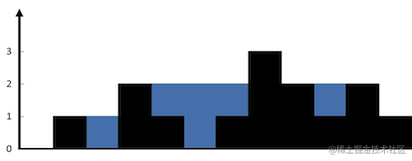``````输入：height = [0,1,0,2,1,0,1,3,2,1,2,1]

``````输入：height = [4,2,0,3,2,5]

• n == height.length
• 0 <= n <= 3 * 104
• 0 <= height[i] <= 105

• 如果这个较短的柱子的长度大于当前柱子，那么雨水的深度就是较短的柱子减去当前柱子的长度；
• 如果这个较短的柱子的长度小于等于当前柱子，那么雨水的深度就是0。

``````/**
* @param {number[]} height
* @return {number}
*/
var trap = function(height) {
let len = height.length, sum = 0
for(let i = 0; i < len - 1; i++){
// 计算当前柱子左侧的最大值
let left = 0
for(let j = i - 1; j >= 0; j--){
left = Math.max(height[j], left)
}
// 计算当前柱子右侧的最大值
let right = 0
for(let j = i + 1; j < len; j++){
right = Math.max(height[j],right)
}
// 计算当前柱子能接的雨水量
if(min > height[i]){
sum += Math.min(left, right) - height[i]
}
}
return sum
};

• 时间复杂度：O(n)，其中 n 是数组 height 的长度。需要遍历两次height数组；
• 空间复杂度：O(1)。

#### （2）爬楼梯

``````输入： 2

1.  1 阶 + 1 阶
2.  2 阶

``````输入： 3

1.  1 阶 + 1 阶 + 1 阶
2.  1 阶 + 2 阶
3.  2 阶 + 1 阶

• 第一级台阶：1种方法
• 第二级台阶：2种方法
• 第n级台阶：从第n-1级台阶爬一级，或从第n-2级台阶爬2级

``````/**
* @param {number} n
* @return {number}
*/
var climbStairs = function(n) {
const dp = []
dp = 1
dp = 1
for(let i = 2; i <= n; i ++){
dp[i] = dp[i - 1] + dp[i - 2]
}
return dp[n]
};

``````/**
* @param {number} n
* @return {number}
*/
var climbStairs = function(n) {
let a = 1, b = 1, res = 1;
for (let i = 1; i < n; i++) {
a = b
b = res
res = a + b
}
return res
};

• 时间复杂度：O(n2)，递归树的深度为n，所以时间复杂度为O(n2)；
• 空间复杂度：O(n)，这里需要初始化一个数组用来保存每一层台阶的方法数，有n个数，所以空间复杂度为O(n)；

• 时间复杂度：O(n)，需要循环执行n次，所以时间复杂度为O(n)；
• 空间复杂度：O(1)，这里只用了常数个变量作为辅助空间，所以空间复杂度为 O(1)；

#### （3）最大正方形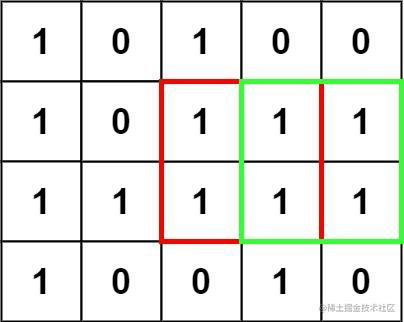``````输入：matrix = [["1","0","1","0","0"],["1","0","1","1","1"],["1","1","1","1","1"],["1","0","0","1","0"]]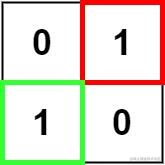``````输入：matrix = [["0","1"],["1","0"]]

``````输入：matrix = [["0"]]

• `m == matrix.length`
• `n == matrix[i].length`
• `1 <= m, n <= 300`
• `matrix[i][j]``'0'``'1'`

• 如果当前的值为0，此时该点不存在于正方形中，直接给dp[i][j]赋值为0；
• 如果当前的值为1，dp[i][j]的值由其上、左、左上的三个值的最小值决定，所以其状态转移方程是：
``````dp[i][j] = Math.min(dp[i - 1][j], dp[i][j - 1], dp[i - 1][j - 1]) + 1

``````/**
* @param {character[][]} matrix
* @return {number}
*/
var maximalSquare = function(matrix) {
const m = matrix.length, n = matrix.length
let res = 0
if(!matrix || m === 0 || n === 0){
return 0
}

let dp = new Array(m)
for(let i = 0; i < m; i++){
dp[i] = new Array(n).fill(0)
}

for(let i = 0; i < m; i++){
for(let j = 0; j < n; j++){
if(matrix[i][j] === '1'){
if(i === 0 || j === 0){
dp[i][j] = 1
}else{
dp[i][j] = Math.min(dp[i - 1][j], dp[i][j - 1], dp[i - 1][j - 1]) + 1
}
res = Math.max(dp[i][j], res)
}
}
}
return res * res
};

• 时间复杂度：O(mn)，其中 m 和 n 是二维矩阵的行数和列数。我们需要遍历二维矩阵中的每个元素来计算 dp 的值。
• 空间复杂度：O(mn)，其中 m 和 n 是二维矩阵的行数和列数。我们创建了一个和原始矩阵大小相同的数组 dp 来保存当前正方形的最大边长。